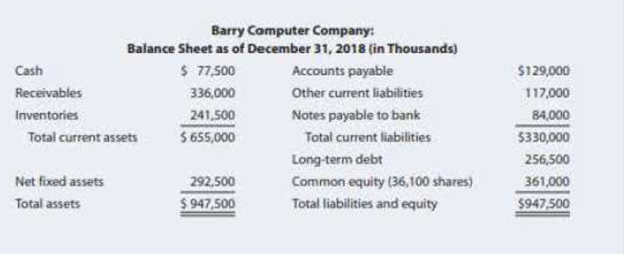Chapter 4, Problem 23PFundamentals of Financial Manageme...

15th Edition
Eugene F. Brigham + 1 other
ISBN: 9781337395250

Solutions

Chapter
SectionFundamentals of Financial Manageme...

15th Edition
Eugene F. Brigham + 1 other
ISBN: 9781337395250
Textbook Problem

RATIO ANALYSIS Data for Barry Computer Co. and its industry averages follow. The firm’s debt is priced at par, so the market value of its debt equals its book value. Since dollars are in thousands, number of shares are shown in thousands too. a. Calculate the indicated ratios for Barry. b. Construct the DuPont equation for both Barry and the industry. c. C Outline Barry’s strengths and weakness as revealed by your analysis. d. Suppose Barry had doubled its sales as Well as its inventories, accounts receivable and common equity during 2018. How would that information affect the validity of your ratio analysis? (Hint: Think about averages and the effects of rapid growth on ratios if averages are not used. No Calculated are needed.)Barry Computer Company: Income Statement for Year Ended December 31, 2018 (in Thousands) Sales   $1,607,500 Cost of goods sold Materials$ 717,000   Labor 453,000   Heat, light, and power 68,000   Indirect labor 113,000   Depreciation 41,500 1,392,500 Gross profit   $215,000 Selling expenses 115,000 General and administrative expenses 30,000 Earnings before interest and taxes (EBIT)$ 70,000 Interest expense   24,500 Earnings before taxes (EBT)   $45,500 Federal and State income taxes (40%) 18,200 Net income$ 27,300 Earnings per share   0.75623 Price per share on December 31, 2018   \$ 12.00 Ratio Barry Industry Average Current _____ 2.0× Quick _____ 1.3× Days sales outstanding _____ 35 days Inventory Turnover _____ 6.7× Total assets turnover _____ 3.0× Profit margin _____ 1.2% ROA _____ 3.6% ROE _____ 9.0% ROIC _____ 7.5% TIE _____ 3.0× Debt/Total capital _____ 47.0% M/B _____ 4.22 P/E _____ 17.86 EV/EBITDA _____ 9.14 *Calculation is based on a 365-day year.

a.

Summary Introduction

To Determine: The indicated ratios for Company BCC.

Introduction: Ratio analysis is the procedure of analysing and contrasting financial data by ascertaining important financial statement numerical calculations as opposed to looking at details from each financial statement.

Explanation

Determine the indicated ratios for Company BCC

Using a excel spreadsheet the ratios are determined as the below,

Excel Workings:

b.

Summary Introduction

To Determine: The DuPont equation for Company BCC and industry.

c.

Summary Introduction

To Determine: The strength and weakness of Company BCC.

d.

Summary Introduction

To Determine: The reasons on the information affecting the validity of ratio analysis.

Still sussing out bartleby?

Check out a sample textbook solution.

See a sample solution

The Solution to Your Study Problems

Bartleby provides explanations to thousands of textbook problems written by our experts, many with advanced degrees!

Get Started

Find more solutions based on key concepts